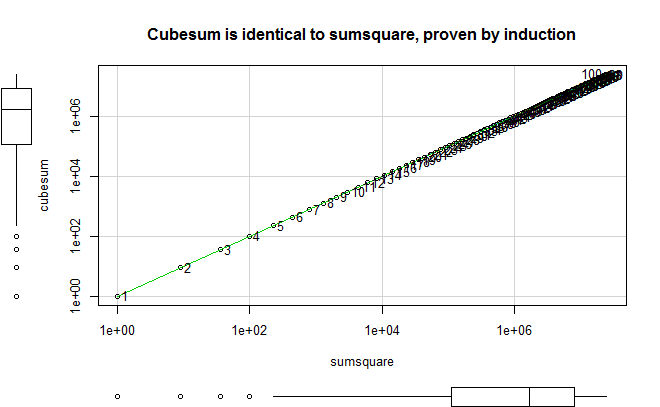R can tell us:

```DF.numbers = data.frame(cubesum=numeric(),sumsquare=numeric()) #initial dataframe
for (n in 1:100){ #loop and fill in
DF.numbers[n,"cubesum"] = sum((1:n)^3)
DF.numbers[n,"sumsquare"] = sum(1:n)^2
}

library(car) #for the scatterplot() function
scatterplot(cubesum ~ sumsquare, DF.numbers,
smoother=FALSE, #no moving average
labels = rownames(DF.numbers), id.n = nrow(DF.numbers), #labels
log = "xy", #logscales
main = "Cubesum is identical to sumsquare, proven by induction")

#checks that they are identical, except for the name
all.equal(DF.numbers["cubesum"],DF.numbers["sumsquare"], check.names=FALSE)```One can increase the number in the loop to test more numbers. I did test it with 1:10000, and it was still true.